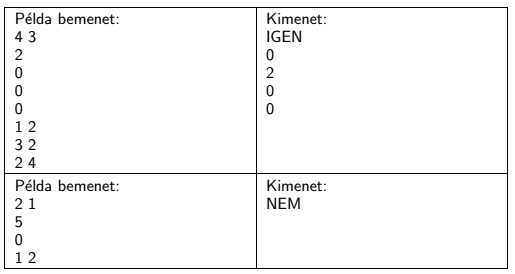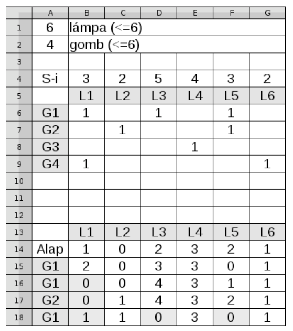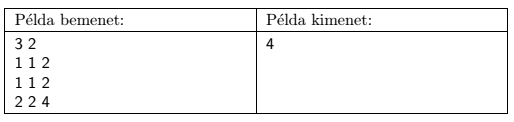Mathematical and Physical Journal
for High Schools
Issued by the MATFUND Foundation
 Already signed up? New to KöMaL?

# KöMaL Problems in Informatics, December 2014

Show/hide problems of signs:## Problems with sign 'I'

Deadline expired on January 12, 2015.

I. 361. The disc shooting game'' is a single-person game played on a simple graph on $\displaystyle N$ vertices ($\displaystyle 1\le N\le 20$) in the following way. Initially some discs are placed on every vertex. In a given step the player selects an arbitrary vertex, and if the number of discs on that vertex is greater than or equal to the number of neighbors of the vertex, then the player distributes one disc from the vertex to each neighbor (two vertices are neighbors if they are directly connected by an edge). The player wins if no such distribution is possible. Your task is to determine, for a given initial state, whether or not the player can win. You can use and assume the following hints: $\displaystyle (i)$ if it is possible for the player to win, then the vertices can be selected in an arbitrary order, and - sooner or later - the game will end; $\displaystyle (ii)$ if the game is still played after 1000 steps, then you should display a CANNOT WIN message.

Your program should read $\displaystyle (i)$ the values of $\displaystyle N$ and $\displaystyle M$ (the number of vertices) from the first line of the standard input; $\displaystyle (ii)$ the number of discs initially placed on the vertices from the following $\displaystyle N$ lines; $\displaystyle (iii)$ finally, the graph description from the last $\displaystyle M$ lines: each such line contains two numbers, $\displaystyle a$ and $\displaystyle b$, meaning that vertex $\displaystyle a$ and vertex $\displaystyle b$ are neighbors. Your program should write the message CANNOT WIN to the first line of the standard input if the game does not end in 1000 steps as given in the above hint. If the game ends, then a CAN WIN message should appear, together with the number of discs on each vertex in the final state in the following $\displaystyle N$ lines (by using the same vertex ordering as in the input).

In the table two sample inputs (Példa bemenet'') and their corresponding output (Kimenet'') are presented, with IGEN meaning CAN WIN, and NEM meaning CANNOT WIN.The source code (i361.pas, i361.cpp, ...) without the .exe or any other auxiliary files generated by the compiler but with a short documentation (i361.txt, i361.pdf, ...), also describing which developer environment to use for compiling the source, should be submitted in a compressed file i361.zip.

(10 pont)

solution, statistics

I. 362. Let us consider the following configuration of $\displaystyle L~(\le 6)$ lamps and $\displaystyle G~(\le 6)$ buttons controlling this system. Each lamp has a counter. The on/off status of the $\displaystyle i$th lamp is reversed as soon as its counter reaches the value $\displaystyle S_{i}$, and in this case, the new value of this counter will be 0. When pressing the $\displaystyle j$th button, we always increase the same set of lamp counters by 1. Each lamp is controlled by at least one button, and similarly, each button controls at least one lamp.

The operation of this system is simulated by using a spreadsheet application. In the example, lámpa'' is lamp, gomb'' is button, and the row Alap'' describes the initial state of the system.$\displaystyle \bullet$ the number of lamps and buttons are entered in cells A1 and A2, respectively;

$\displaystyle \bullet$ in rows 5 and 13, the header row content is displayed in a cell only if the corresponding lamp is present;

$\displaystyle \bullet$ in column A and beginning with row 6, the header column is displayed in a cell only if the corresponding button is present;

$\displaystyle \bullet$ the counter values - to change the state of the lamp when this value is reached - are entered in row 4;

$\displaystyle \bullet$ the relation between the lamps and buttons is entered in the range beginning with cell B6;

$\displaystyle \bullet$ the initial state of the counters is entered in row 14, and we assume that initially no lamp is on;

$\displaystyle \bullet$ beginning with cell A15 and upon entering a button number in the same column one by one, the corresponding row should display the status of the counters, and a text message or a background color change should show when the corresponding lamp is on.

When creating your table, you should use conditional formatting and functions to make sure that cell values are displayed only when necessary, and wrong or missing cell values are clearly visible (A1:A2, B4:G4, A15:A114, B6:G11). Your table should handle at least 100 button presses. (We remark that conditional formatting is not a requirement in the matriculation examination.)

In your solution you cannot use any macros, however, an arbitrary number of auxiliary cells may be used to the right of column H.

Your sheet (i362.xlsx, i362.ods,...) containing the simulation, together with a short documentation (i362.txt, i362.pdf) and also describing the name and version number of the spreadsheet application, should be submitted in a compressed file (i362.zip).

(10 pont)

solution, statistics

I. 363. The Foxes and the Rabbit'' game is a simple and quick game played on a chessboard by two players. One player controls the foxes, the other player controls the rabbit, and only the white squares of the board are used in the game. Initially, four identical black pieces (the foxes'') are placed in the first row of the board. The other player then freely positions a white piece (the rabbit'') in the last row. The players take turns, and move one of their pieces diagonally to a free neighboring square. The foxes start the game. A fox can move only forward, but there is no such restriction on the rabbit moves. The foxes win if the rabbit is unable to move, and the rabbit wins if it gets behind the foxes.

You should create the above game that can be played also offline. Your program should make sure that the players do not violate the rules. The steps of the game should be visible and recorded in a separate window, from where we can copy them at the end of the game. Moreover, given a sequence of steps, your program should be able to replay an earlier game by using an animation that can be followed easily. In this case, it is not necessary to check whether the given steps are valid.

To solve the task, you can use any programming environment. Your program should be pleasant to look at and easy to use. Due to the required animations, programs that use only text mode will not be considered as complete.

The description of your work (i363.pdf), containing the main steps of the solution and information on how to interpret the notation used to record the steps of a game, together with the source code of your program, and any files necessary to compile and run the program should be submitted in a compressed file i363.zip.

(10 pont)

solution, statistics## Problems with sign 'S'

Deadline expired on January 12, 2015.

S. 94. Some researchers discovered that the results of a particular animal experiment tend to be unreliable when too many animals get in contact with one another. At present there are too many animals in a square field of size $\displaystyle N\times N$ ($\displaystyle 2\le N\le 17$). We want to build $\displaystyle K$ ($\displaystyle 1\le K\le 2N-2$) fences to separate them. A fence always runs parallel with one of the field edges, and the distance between them is an integer. Moreover, a fence passes through the square completely, that is, a fence cannot end in the square interior. Given the above constraints, by suitably building fences we would like to minimize the maximum number of animals in any connected part.

Your program should read the values of $\displaystyle N$ and $\displaystyle K$ ($\displaystyle 2\le N\le 17$, $\displaystyle 1\le K\le 2N-2$) from the first line of the standard input; then $\displaystyle N$ integers from each of the following $\displaystyle N$ lines, describing for each row the number of animals in each of the $\displaystyle N$ unit squares of that row. The first and only line of the standard output should contain the maximum number of animals that are not separated from each other, provided that the best fence-building strategy was used.

In the example, Példa bemenet'' is a sample input, and Példa kimenet'' is the corresponding output.Explanation: we have to build two fences. We build the first fence between the 2nd and the 3rd columns, and the second fence between the 2nd and the 3rd rows. Then the upper left area will contain $\displaystyle 1+1+1+1=4$ animals, the upper right area will have $\displaystyle 2+2=4$, the lower left area $\displaystyle 2+2=4$, and the lower right area will have 4 animals, so we display 4 as an output.

Scoring and bounds. You can obtain 1 point for a brief and proper documentation clearly describing your solution. Nine further points can be obtained provided that your program solves any arbitrary valid input within 1 second of running time.

The source code (s94.pas, s94.cpp, ...) without the .exe or any other auxiliary files generated by the compiler but with a short documentation (s94.txt, s94.pdf, ...), also describing which developer environment to use for compiling the source, should be submitted in a compressed file s94.zip.

(10 pont)

solution, statistics

\$Var(Body)